## Section5Polynomial Regression

### Subsection5.1Introduction

Suppose we want to apply regression or classification techniques to data sets as in Figure 5.1.

###### Question5.2.
Will linear regression be a good choice for the regression data? Will logistic regression be a good choice for the classification data? Why or why not? Answer
Probably not! Both models have a linear component either for the model itself, as in linear regression, or for the decision boundaries, as in logistic regression. They are not likely to work well on these data sets that do not appear linear!

###### Question5.3.
Where should we make changes in our six step summary?
1. Process Data for $X$ and $y\text{.}$

2. Learn Function $F$ such that $F(X) \approx y \text{.}$

3. Choose a Model.

4. Choose a Cost Function.

5. Solve for $\Theta\text{.}$

6. Make Predictions!

For polynomial regression we are going to allow the model to have a polynomial component instead of simply a linear component. In theory, changing “Step 3: Choose a Model” is the only change we are going to make. However, in implementation we are actually going to modify $X$ by adding polynomial versions of our features. So in practice, we are only going to change “Step 1: Process the Data”. Its a little bit sneaky, but then we don't have to change any other steps in the model.

###### Example5.4.One Feature.

Suppose we have one feature, $x_1\text{.}$ Then our linear regression model would have

\begin{equation*} F(X)=\theta_0 + \theta_1 x_1 \end{equation*}

we could convert this to allowing a polynomial of degree 3 by

\begin{equation*} F(X_p)=\theta_0 +\theta_1 x_1 +\theta_2 x_1^2 + \theta_3 x_1^3\text{.} \end{equation*}

Note that $F$ is not linear in $x_1\text{,}$ but it is still linear in $\Theta\text{.}$

###### Question5.5.
We can rewrite as
\begin{equation*} F(X_p) = [1, x_1, x_1^2, x_1^3] * \Theta = \left[ \begin{matrix} \theta_0 \\ \theta_1 \\ \theta_2 \\ \theta_3 \\ \end{matrix} \right]\text{.} \end{equation*}
Thus, we can (and will!) think about polynomial regression as linear regression (or logistic regression) but on more features. That is, we will change

\begin{equation*} X= \left[ \begin{matrix} 1 \amp x_1^{(1)} \\ 1 \amp x_1^{(2)} \\ \amp \vdots \\ 1 \amp x_1^{(m)} \\ \end{matrix} \right] \text{ and } \Theta = \left[ \begin{matrix} \theta_0 \\ \theta_1 \\ \end{matrix} \right] \end{equation*}

to

\begin{equation*} X_p= \left[ \begin{matrix} 1 \amp x_1^{(1)} \amp \left(x_1^{(1)}\right)^2 \amp \left(x_1^{(1)}\right)^3 \\ 1 \amp x_1^{(2)} \amp \left(x_1^{(2)}\right)^2 \amp \left(x_1^{(2)}\right)^3 \\ \amp \amp \vdots \amp \\ 1 \amp x_1^{(m)} \amp \left(x_1^{(m)}\right)^2 \amp \left(x_1^{(m)}\right)^3 \\ \end{matrix} \right] \text{ and } \Theta_p = \left[ \begin{matrix} \theta_0 \\ \theta_1 \\ \theta_2 \\ \theta_3 \\ \end{matrix} \right] \end{equation*}

Note that we are only adding polynomial terms to our features and not to our bias term, 1. A constant to any power is still a constant after all.

Hmmm. We increased from 2 to 4 $\Theta$ parameters. What do we think about that?

###### Question5.6.
How many $\Theta$ parameters would there be if there is one feature and a degree 10 polynomial? One feature and a degree $d$ polynomial? Answer
11 or $d+1$ in general.
###### Example5.7.Multiple Features.

Its more complicated with more features because we need to remember all the cross terms between features. For a data set with two features we want to change

\begin{equation*} F(X)=\theta_0 + \theta_1 x_1 +\theta_2 x_2 \end{equation*}

into a polynomial of degree 3 by

\begin{equation*} F(X_p)=\theta_0 +\theta_1 x_1 +\theta_2 x_2 + \theta_3 x_1^2 +\theta_4 x_2 ^2 +\theta_5 x_1 x_2 + \theta_6 x_1^3 + \theta_7 x_2^3 + \theta_8 x_1 x_2^2 +\theta_9 x_1^2 x_2 \text{.} \end{equation*}

Again, note that $F$ is not linear in $X_p\text{,}$ but it is still linear in $\Theta\text{.}$ Thus, we can (and will!) think about polynomial regression as linear regression (or logistic regression) but on more features. So we will change

\begin{equation*} X= \left[ \begin{matrix} 1 \amp x_1^{(1)} \amp x_2^{(1)} \\ 1 \amp x_1^{(2)} \amp x_2^{(2)}\\ \amp \vdots \amp \\ 1 \amp x_1^{(m)} \amp x_2^{(m)} \\ \end{matrix} \right] \text{ and } \Theta = \left[ \begin{matrix} \theta_0 \\ \theta_1 \\ \theta_2 \\ \end{matrix} \right] \end{equation*}

to

\begin{equation*} X_p= \left[ \begin{matrix} 1 \amp x_1^{(1)} \amp x_2^{(1)} \amp \left(x_1^{(1)}\right)^2 \amp \left(x_2^{(1)}\right)^2 \amp x_1^{(1)}x_2^{(1)} \amp \left(x_1^{(1)}\right)^3 \amp \left(x_2^{(1)}\right)^3 \amp x_2^{(1)}\left(x_1^{(1)}\right)^2 \amp x_1^{(1)}\left(x_2^{(1)}\right)^2 \\ 1 \amp x_1^{(2)} \amp x_2^{(2)} \amp \left(x_1^{(2)}\right)^2 \amp \left(x_2^{(2)}\right)^2 \amp x_1^{(2)}x_2^{(2)} \amp \left(x_1^{(2)}\right)^3 \amp \left(x_2^{(2)}\right)^3 \amp x_2^{(2)}\left(x_1^{(2)}\right)^2 \amp x_1^{(2)}\left(x_2^{(2)}\right)^2 \\ \amp \amp \amp \amp \amp \vdots \amp \amp \amp \amp \\ 1 \amp x_1^{(m)} \amp x_2^{(m)} \amp \left(x_1^{(m)}\right)^2 \amp \left(x_2^{(m)}\right)^2 \amp x_1^{(m)}x_2^{(m)} \amp \left(x_1^{(m)}\right)^3 \amp \left(x_2^{(m)}\right)^3 \amp x_2^{(m)}\left(x_1^{(m)}\right)^2 \amp x_1^{(2m)}\left(x_2^{(m)}\right)^2 \\ \end{matrix} \right] \text{ and } \Theta_p = \left[ \begin{matrix} \theta_0 \\ \theta_1 \\ \theta_2 \\ \theta_3 \\ \theta_4 \\ \theta_5 \\ \theta_6 \\ \theta_7 \\ \theta_8 \\ \theta_9 \\ \end{matrix} \right] \end{equation*}

Hmmm. We increased from 3 to 10 $\Theta$ parameters. What do we think about that?

###### Question5.8.
How many $\Theta$ parameters would there be if there are two features and a degree 4 polynomial? Answer
We need all the terms we have for two features and a degree 3 polynomial, but we need to add all possible ways to get a degree 4 polynomial. $x_1^4, x_1^3x^1, x_1^2x^2, x_1^1x^3, x_1^1x^3$ so there are $10+5=15$ parameters here.

###### Question5.9.
How many $\Theta$ parameters are there if we have 3 features and use a degree 5 polynomial? What about $f$ features and use a degree $d$ polynomial? Answer
Let's write them all down. Ack! Let's not. There are 56 parameters here. In general the number of $\Theta$ parameters given $f$ features and a degree $d$ polynomial is
\begin{equation*} \frac{(f+d)!}{d!\,f!}\text{.} \end{equation*}
Hmmm. What do we think about that?
###### Question5.10.
Where does that formula come from? Hint
This is an excursion into combinatorics. So feel free to skip. However, for those interested in the mathematics behind this,
\begin{equation*} \frac{(f+d)!}{d!\,f!} \end{equation*}
is the formula for the number of ways to choose $f$ objects from a set of $f+d$ objects. This is sometimes written as $C(f+d,f)=\frac{(f+d)!}{d!\,f!}\text{.}$ So we can understand this formula by figuring out a way to choose $f$ objects from a set of $f+d$ objects that corresponds to the terms we need to produce in our matrix $X\text{,}$ that is all the combinations of powers of $x_1, x_2, \cdots x_f$ that have degree $d$ or less. Can you think of a way to do this? Solution
The most obvious set of $f+d$ objects is $S= \{ 1,2,3, \cdots, d, X_1, X_2, \cdots X_f \}$ where $d$ is the degree of the polynomial and $X_i$ is one of the $f$ features. We need to choose $f$ objects from set $S$ and from those $f$ objects construct an $X_1^{a_1} X_2^{a_2} \cdots X_f^{a_f}$ where $a_1 + a_2 + \cdots a_f \leq d\text{.}$ Its a bit of work to think of the right way to construct combinations of powers of $x_1, x_2, \cdots x_f$ that have degree $d$ or less. But here's the idea.
1. For each $X_i$ that we chose from $S\text{,}$ we set the power of $X_i$ to zero.
2. For the first number, $u$ that we chose from $S\text{,}$ we consider the $X_j$s for which we have not already determined a power and pick the one with the smallest $j$ and set its power to $u\text{.}$
3. For the second number, $v$ that we chose from $S\text{,}$ we consider the $X_j$s for which we have not already determined a power and pick the one with the smallest $j$ and set its power to $v-u\text{.}$
4. For the third number, $w$ that we chose from $S\text{,}$ we consider the $X_j$s for which we have not already determined a power and pick the one with the smallest $j$ and set its power to $w-v-u\text{.}$
5. Similarly, continue this process for each number that is chosen.
Let's go back to our example of $f=3$ and $d=5$ and outline each of the cases for combinations of numbers and features. The set $S= \{ 1,2,3,4,5,X_1,X_2,X_3 \} \text{.}$ We need to choose three objects and from those three objects construct an $X_1^aX_2^bX_3^c$ where $a+b+c \leq 5$
1. Case 1. Suppose we pick three $X_i\text{;}$ that is we pick $X_1, X_2, X_3\text{.}$ We assign this to $X_1^0X_2^0X_3^0=1\text{.}$ So we have the bias term.
2. Case 2. Suppose we pick two $X_i$ and one number; that is we pick $X_i, X_j$ and a number $u\text{.}$ We assign this to $X_i^0X_j^0X_k^u=X_k^u$ where $k \in \{1,2,3\}$ and $k \neq i\text{,}$$k \neq j\text{.}$ In this case, we have all powers of a single feature.
3. Case 3. Suppose we pick one $X_i$ and two numbers; that is we pick $X_i$ and number $u,v\text{.}$ We assign this to $X_i^0X_j^uX_k^{v-u}=X_j^uX_k^{v-u}$ where $j \lt k$ and $j,k \in \{1,2,3\}$ and $j \neq i\text{,}$$k \neq i\text{.}$ In this case, we have all powers of two features.
4. Case 4. Suppose we pick three numbers; that is we pick number $u,v,w\text{.}$ We assign this to $X_i^uX_j^{v-u}X_k^{w-v-u}$ where $i \lt j \lt k \text{.}$ For three features, this must be $X_1^uX_2^{v-u}X_3^{w-v-u}$ In this case, we have all powers of all three features.
We thus see that the number of $\Theta$ parameters should indeed correspond to
\begin{equation*} C(8,3)=\frac{(8)!}{3!\,5!}=56. \end{equation*}

### Subsection5.2Implementation

In the previous section we saw that the idea for implementing polynomial regression is to

1. Add polynomial features to our data X
2. Perform Linear Regression (or Logistic Regression) on the enhanced data set X.

We already know how to implement Linear and Logistic Regression, so we just need to know how to add polynomial features to our data. We will implement this with the PolynomialFeatures() tool from sklearn.


from sklearn.preprocessing import PolynomialFeatures
poly_features = PolynomialFeatures(degree=3, include_bias=False)
X_poly = poly_features.fit_transform(X)


In this case, $X$ is our original data set, degree=3 specifies the degree of the polynomial model, and include_bias=True will add the column of ones, include_bias=False does not. (Usually we do not need to add the column of ones because the ML algorithms in sklearn will do this for us.)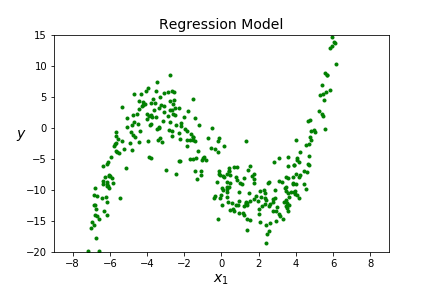###### Question5.11.
How many features in this dataset? Answer
Just one!
###### Question5.12.
Is this discrete or continuous data? Answer
Continuous
###### Question5.13.
What type of ML algorithm should we use? Answer
We would like to try polynomial regression! Remember we will implement this by by combining PolynomialFeatures() and LinearRegression().

###### Question5.14.
How does it look? What important steps did we skip? Answer
Looks ok, but we didn't separate the data into training and testing or scale the data yet!
###### Question5.15.
How important is scaling the data in polynomial regression? Answer
How do the sizes of $x$ and $x^3$ compare? Generally, they are quite different! So scaling is really important here!

We now have multiple preprocessing steps that we need to apply to transform our data, first on training data and then on testing data. It can get complicated with so many steps, so we are going to use a tool from sklearn called Pipeline. Pipeline allows us to sequentially compose a list of transformations and conclude with an estimator. For us the transformations will be the data processing steps and the estimator will be the machine learning algorithm. Pipeline will call .fit_tranform on the list of transformations in the order they are listed, and will call .fit on the last estimator. The main parameter for Pipeline(steps, memory=None, verbose=False) is steps which is a list of (name,transform) tuples. To apply the steps

1. Add polynomial features to data set
2. Apply linear regression

we would create a pipeline with

from sklearn.pipeline import Pipeline
poly_model  = Pipeline([
('poly_feat', PolynomialFeatures(degree=3, include_bias=False)),
('lin_reg', LinearRegression()),
])


We interact with poly_model in the same way we would interact with LinearRegression(), or whatever the last item in our steps is. That is, we can create the model with poly_model.fit(X,y), apply the model with poly_model.predict(X) and score the model with poly_model.score(X,y).

###### Question5.16.
What will poly_model.fit(X,y) do? What will poly_model.predict(X) do? What will poly_model.score(X,y) do? Answer
1. poly_model.fit(X,y) first calls PolynomialFeatures.fit_transform(X) and then calls LinearRegression.fit(X,y). So that it both creates the polynomial features and applies linear regression to the polynomial features to model the data.
2. poly_model.predict(X) first calls PolynomialFeatures.fit_transform(X) and then calls LinearRegression.predict(X).
3. poly_model.predict(X) first calls PolynomialFeatures.fit_transform(X) and then calls LinearRegression.score(X).

We can still access of the individual steps if we want, but it takes a bit more work. We method uses the named_steps attribute of the Pipeline. For example, poly_model.named_steps.lin_reg.intercept_ will give the intercept from the Linear Regression model. We can also access this by with poly_model['lin_reg'].intercept_.

Let's repeat our previous code using a Pipeline! Not we still haven't split into training and testing data yet, or applied feature scaling.

###### Question5.17.
What does intercept [-7.5258753] and coefficients [[-3.76629704 0.30092201 0.15046627]]. tell us about $\Theta\text{?}$ Answer
Note these are arrays rather than values, so be careful about how you call the values rather than the array. The values in the arrays give us the coefficents of the cubic $\Theta = -7.526 -3.766 x_1 + 0.301 x_1^2+ 0.150 x_1^3.$

In general, the process we want for a real data set would be

1. Take a look at the data and make sure you understand it.
2. SPLIT the data (both features and labels) into Training and Testing Sets. (Eventually swap steps 1 and 2.)
3. ADD relevant features to data set. (For example, add polynomial features).
4. SCALE the FEATURES of the TRAINING data (fit_transform).
5. TRAIN the model (fit) using the TRAINING data. (For example, apply linear regression.)
6. TEST the model using the TESTING data.
7. Experiment - How did your model do? Can you do better?

The last step is really important. Experimenting with the model is always part of your job as a data scientist!

###### Question5.18.
For polynomial regression, which of these steps should be combined into a Pipeline and how would we do it? Answer
We can combine polynomial regression, feature scaling and linear regression into a Pipeline.
from sklearn.pipeline import Pipeline
poly_model  = Pipeline([
('poly_feat', PolynomialFeatures(degree=3, include_bias=False)),
('std_scaler', StandardScaler()),
('lin_reg', LinearRegression()),
])

###### Question5.19.
Why is splitting the data not included in the Pipeline? Answer
We want to apply the steps separately to training and testing data! So we always want to do that first!
We will look at a full example of this in the Jupyter Notebook shortly.

### Subsection5.3Underfitting and Overfitting

Suppose we have the following data set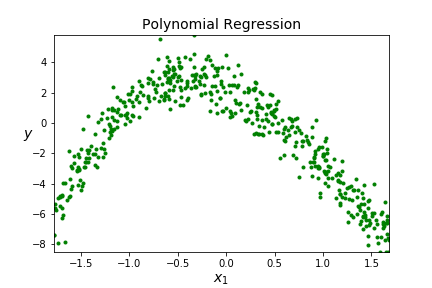and we want to model it with polynomial regression. How do we choose the best degree for the polynomial? We use the built in score function (1 is best possible score) and obtain the the following scores for different degrees.
 degree score 1 0.1566 2 0.8887 3 0.9217 4 4:0.9220 20 20: 0.9246 100 0.9254 125 0.9255 130 0.9200
Should we just pick the best score? More degrees are better, right? Let's look at some pictures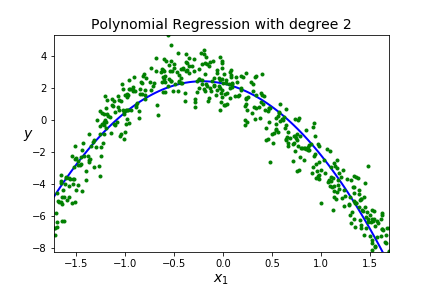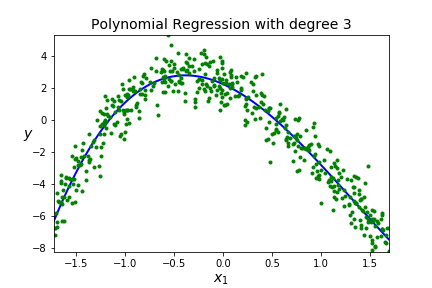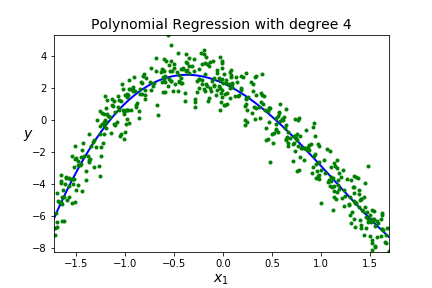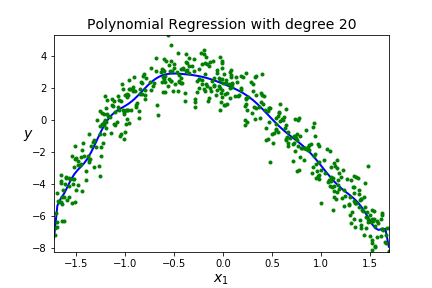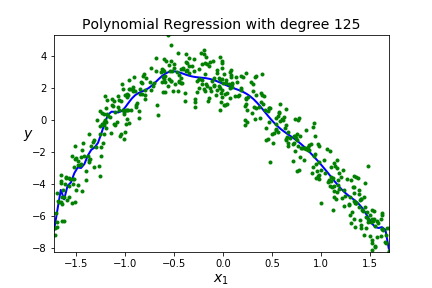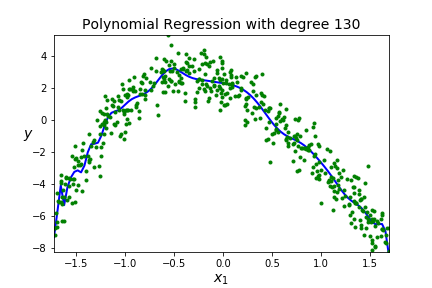Not necessarily, we might overfit the data!

A BIG IDEA - You want to get a model that fits your data well AND generalizes well to new data sets.

Underfitting A model is underfitting if it is not matching the shape of the data, you assumed the wrong shape for $F(x)\text{,}$ aka wrong model. High error when on the training data so the model just doesn't work, it won't be usefull on new data so high error on the testing data. You can see this by poor scores on both the training and testing data.

Overfitting A model is overfitting if it is matching the shape of the training data really well (too well!) It may go through a lot of points on the training data and will have low error on the training data. BUT, you have effectively memorized your data, so this model will not generalize well to new data so high error on the testing data. Scores are good on training data, but poor on testing data.

One way to visualize underfitting versus overfitting is to Plot Learning Curves. We will examine the score on the model as we increase the percentage of training data. Note this is a different use of the term Learning Curves than the Learning Curves from Gradient Descent. There "training" means how many steps of gradient descent we took. We were testing the optimization not the model. Now, we don't want to test how well the optimization is working. Rather, we want to know how our model reacts as it is given more training data.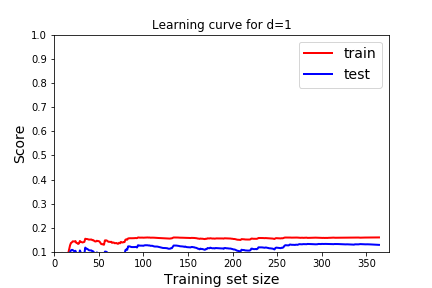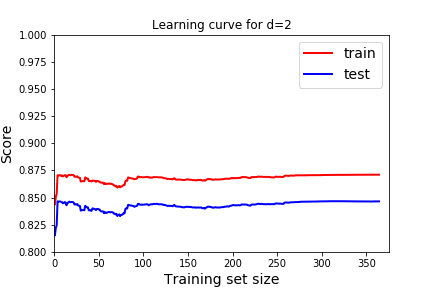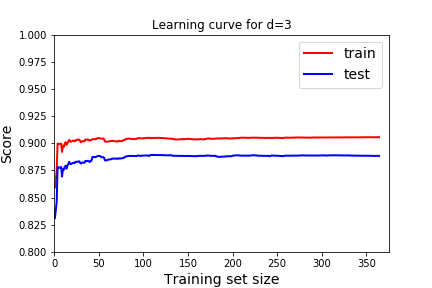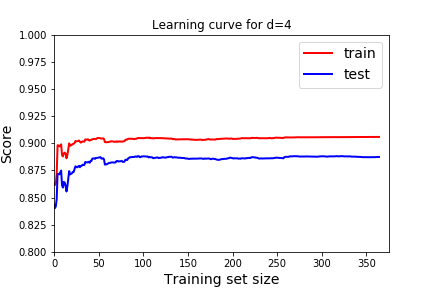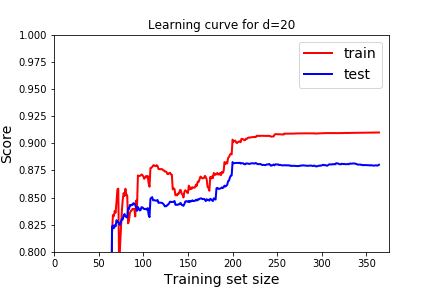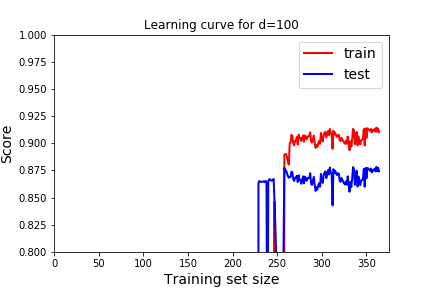What should a learning curve look like for a good model? In all of these, we see that as we train with more data the model gets better at predicting the training data (red), but eventually reaches a plateau.

1. We want the scores to be good on both training and testing. Remember good depends on the score we use. In this case, the R^2 score, 1 indicates a perfect fit, so we want the scores to be close to 1. For the cost function, or mean squared error we would want the scores to be close to 0.
2. We expect the score to be better for the training data than the testing data. But we would like the score on the testing data to be close to the training data.

###### Question5.20.
Which learning curve indicate underfitting and which learning curve indicates overfitting? How would we use these to pick the best degree of the polynomial? How does that usually correspond to degree of the polynomial? Answer
Degree 3 or 4 both look good. Degree 1 has very low scores (note: the scale is different on this graph because the scores are so low). Degree 2 also has lower scores. Underfitting is happening for Degrees 1 and 2 because the scores on the training data aren't very good. For degrees 20 and 100, the score on the training data is very good, but the scores on the testing data are getting worse. Note the gap between training and testing data is increasing. So we'd like there to be little gap between training and testing and for both scores to be good. In general, a large gap indicates overfitting, and bad scores indicate underfitting.

Jupyter notebook for implementation details of this type of learning curves and an example of using polynomial regression with logistic regression.

There are methods for searching the parameter space to find the best polynomial degree. See the next chapter. For now, experiment and get a feel for what these parameters do so that when you use the search features you understand what they are doing. There are also different measures of how well the model is doing. Start with a model, choose a small set of features, question every assumption that you made in your model test each assumption to see if you can approve take good notes for parameters